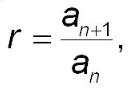# Common Ratio & Common Difference

## What is the Common Ratio?

A common ratio (r) is a non-zero quotient obtained by dividing each term in a series by the one before it. The formula is:.
You could use any two consecutive terms in the series to work the formula. For example, the 2nd and 3rd, 4th and 5th, or 35th and 36th.

## Common Ratio Examples

In a geometric sequence, consecutive terms have a common ratio. For example:

• The series 1, 2, 4, 8, 16, 32, … has an r of 3, because 2/1 = 2, 4/2 = 2, and so on.
• The series 1200, 600, 300, 150, … has an r of ½ because each term is one half of the term before it: 600/1200 = ½, 300/600 = ½…

Watch this one minute video for an example of how to find the common ratio:

## Convergence and Divergence

A geometric series diverges when the absolute value of r is greater to or equal to 1 (|r| ≥ 1); the series converges when r < 1.

## What is the Common Difference

In an arithmetic sequence, the difference (an addition or subtraction) between any two consecutive terms is a constant. This constant is called a common difference. To find the common difference, subtract a term from the one before it. Watch this one minute video for a quick example of how to find the common difference:

## References

University of Toronto Department of Mathematics. Sequences and Series. Retrieved July 10, 2020 from: https://www.math.toronto.edu/preparing-for-calculus/9_sequences/sequences.html

CITE THIS AS:
Stephanie Glen. "Common Ratio & Common Difference" From StatisticsHowTo.com: Elementary Statistics for the rest of us! https://www.statisticshowto.com/common-ratio-common-difference/